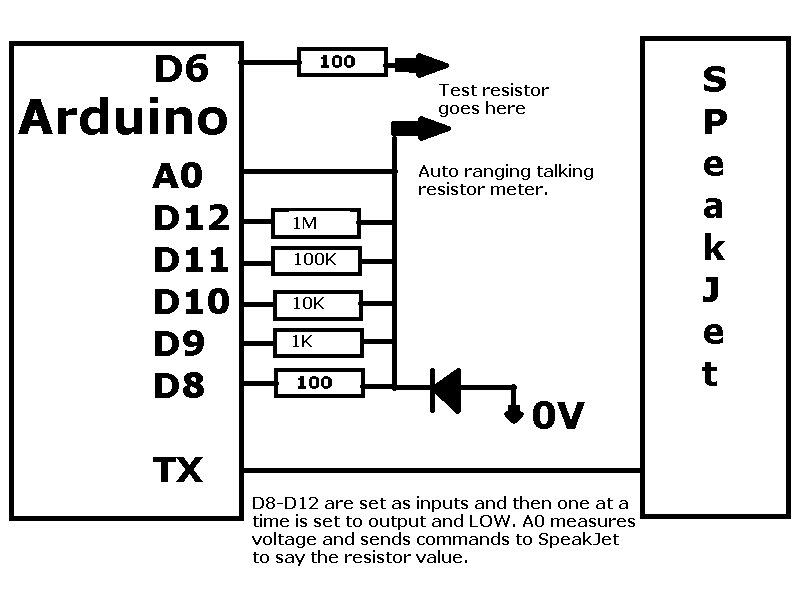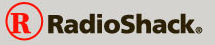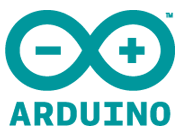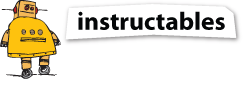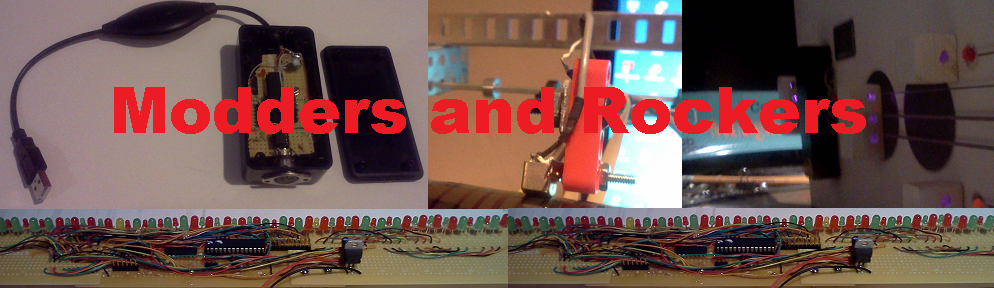# Talking Resistor Meter

On Friday 3/28/14 a friend tweeted

Can someone make an augmented reality iPhone app for color blind people that helps them identify colors? I'd be forever indebted to you
On the drive home I thought up this circuit.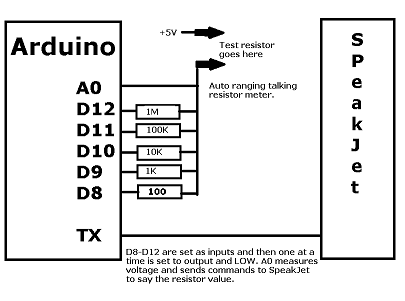It uses an Arduino controller to measure the resistor value and then send commands to the Speakjet to say the value and colors. 4 or 5 resistors are compared to the unknown resistor one at a time. To do this the IO pins on the Arduino are initially set as inputs. As inputs they are high resistance. Then one of the IO pins is set as an output and a LOW value is written. This makes the end of the selected resistor close to 0 volts. The unknown resistor and the selected resistor form a potential divider and the voltage where they join is read using an analog input.

 The talking resistor meter is basically made up of two hardware pieces. An Arduino (UNO or Mega) and a SpeakJet. The Arduino makes the measurements and sends the sounds over a serial link to the SpeakJet that then outputs the speech. The software running on the Arduino is basically broken into two pieces. The measurement part and the talking part. The measurement is done by creating a number of potential dividers where one resistor is known and the other is the unknown one that is being measured.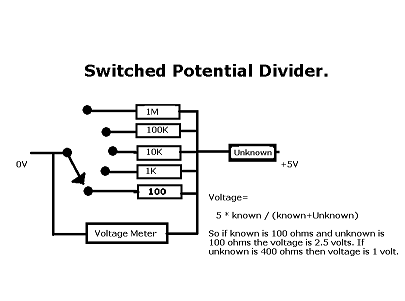## Switching the known resistors

The software tries to find the known resistor that is closest to the unknown one. It does this by selecting each known resistor one at a time and measuring the voltage. The analog input returns a number between 0 and 1024. So the number 512 should mean 2.5 volts. Each known resistor is connected to a digital IO pin. Initially each IO pin is set as an input. As an input it is a high resistance and effectively disconnects the resistor. The one at a time, an IO pin is changed to an output and set LOW. This connects the known resistor to about 0 volts. The analog input is read and then the IO pin is set back to an input. This repeats for each of the known resistors. The measured value that is closest to 512 is used to select the known resistor that is closest to the unknown one.

To use these sources create a folder called res_meter and download the following files into it.

res_meter/res_meter.ino
This is the main source of the talking resistor meter
res_meter/play.ino
This source is used to play a tune. chan 18 is the speakjet.
res_meter/play.h
Defines etc for play.ino.
res_meter/tune.ino
The startup 'tune'.

## Initial design## Updated meter circuit